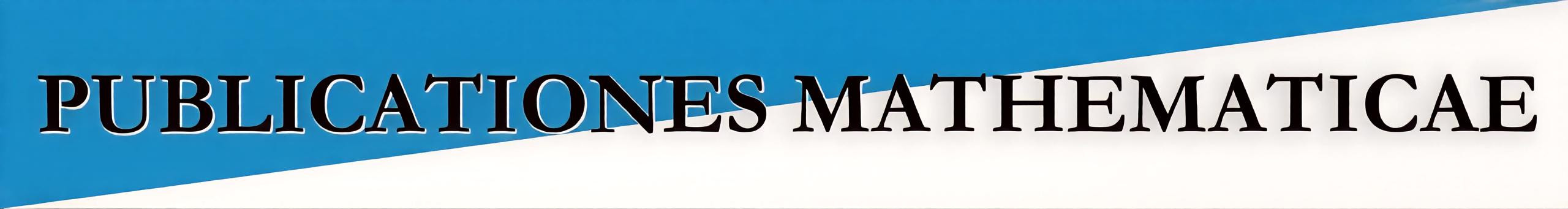﻿ Publicationes MathematicaeIn Print -- Forthcoming Papers Afsaneh Shamsaki and Peyman Niroomand : The classification of nilpotent Lie algebras $L$ when $\dim \mathcal{M}(L)=\dim L^{2}=n-2$ Mikhail Komarov : A lower bound for restricted exponential sums Mohammad Reza Farhangdoost and Ahmad Reza Attari Polsangi : $\mathds{Z}$-graded Hom-Lie superalgebras Alireza Ranjbar-Motlagh : Isometries between spaces of vector-valued differentiable functions II Pingzhi Yuan : Some results on Evans problems Li Lai and Li Zhou : At least two of $\zeta(5), \zeta(7), \ldots, \zeta(35)$ are irrational Mirko D'Ovidio, Anna Chiara Lai and Paola Loreti : Generalized binomials in fractional calculus Anatoly Nazarov, János Sztrik and Anna Kvach : Asymptotic waiting time analysis of finite source $M/GI/1$ retrial queueing systems with conflicts and unreliable server Eszter Gselmann : Polynomial identities satisfied by generalized polynomials Florian Luca, Euloge Tchammou and Alain Togbé : On an exponential Diophantine equation involving powers of consecutive terms of the Padovan sequence Amalendu Ghosh and Dhriti Sundar Patra : On the $m$-quasi-Einstein almost contact manifolds István Mező : New expressions for the Laplace Limit Constant© 2022, Publicationes Mathematicae, Debrecen, Hungary